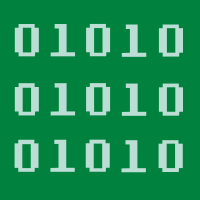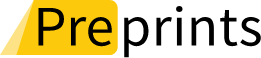## Topic Editors

Prof. Dr. Changyou Wang
College of Applied Mathematics, Chengdu University of Information Technology, Chengdu 610225, China
Prof. Dr. Dong Qiu
College of Science, Chongqing University of Posts and Telecommunications, Chongqing 400065, China
Dr. Yonghong Shen
School of Mathematics and Statistics, Tianshui Normal University, Tianshui 741001, China

# Fuzzy Number, Fuzzy Difference, Fuzzy Differential: Theory and Applications

20 October 2023
20 December 2023
Viewed by
544

## Topic Information

Dear Colleagues,

With the rapid development of new technologies such as artificial intelligence and big date, as the mathematical basis of artificial intelligence, fuzzy set theory has always been concerned by scholars. And fuzzy analysis theory is an important part of it. As the basic concept of fuzzy analysis, fuzzy number, fuzzy difference, fuzzy differential, etc. involve many related important theories, such as: fuzzy difference equation, fuzzy differential equation, fuzzy optimization, fuzzy game, fuzzy decision-making, fuzzy control, etc. Therefore, it is necessary to study the fuzzy number, fuzzy difference, fuzzy differential and related theories and applications.

This Topic, “Fuzzy Number, Fuzzy Difference, Fuzzy Differential：Theory and  Applications”, invites papers on theoretical and applied issues, including, but not limited to, the following:

• Theories and applications of fuzzy Number.
• Theories and applications of fuzzy difference equation.
• Theories and applications of fuzzy differential equation.
• Techniques and algorithms of fuzzy optimization.
• Strategy of fuzzy game.
• Methods and analysis fuzzy decision-making.
• Techniques and methods of fuzzy control.
• This Topic will present the results of research describing recent advances in fuzzy number, fuzzy difference, and fuzzy differential.

Prof. Dr. Changyou Wang
Prof. Dr. Dong Qiu
Dr. Yonghong Shen
Topic Editors

## Keywords

•  fuzzy number
•  fuzzy difference equation
•  fuzzy differential equation
•  fuzzy optimization
•  fuzzy decision-making
•  fuzzy game
•  fuzzy control

## Participating Journals

Journal Name Impact Factor CiteScore Launched Year First Decision (median) APCAlgorithms
algorithms
- 3.3 2008 17.6 Days 1600 CHFAxioms
axioms
1.824 2.6 2012 17.8 Days 1600 CHFInformation
information
- 4.2 2010 21.8 Days 1600 CHFMathematics
mathematics
2.592 2.9 2013 16.8 Days 2100 CHFSymmetry
symmetry
2.940 4.3 2009 14.2 Days 2000 CHFPreprints is a platform dedicated to making early versions of research outputs permanently available and citable. MDPI journals allow posting on preprint servers such as Preprints.org prior to publication. For more details about reprints, please visit https://www.preprints.org.

## Published Papers

This Topic is now open for submission.